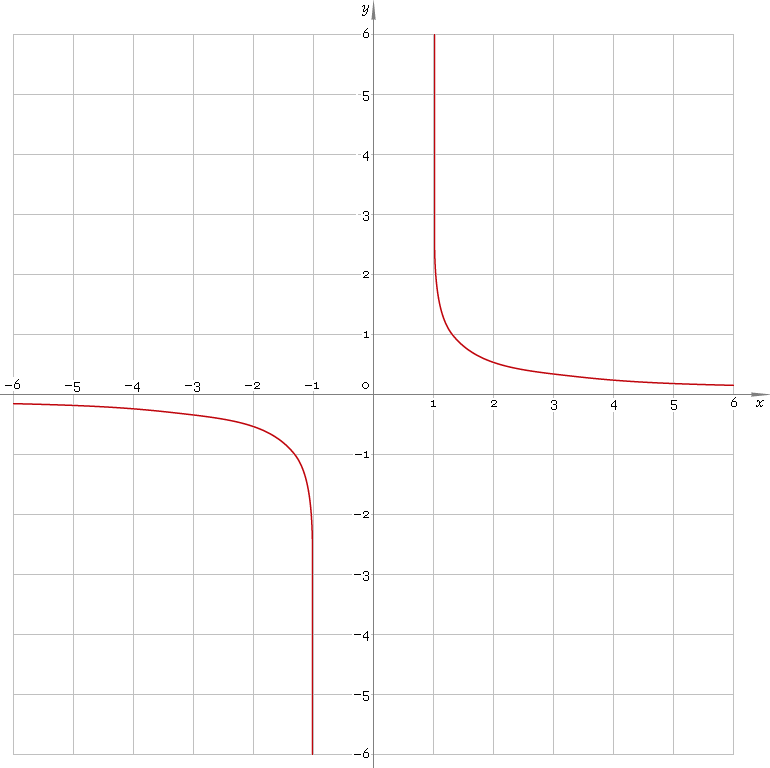The Art of Interface

# arcoth or arcth — arc-hyperbolic cotangent function

Category. Mathematics.

Abstract. Arc-hyperbolic cotangent: definition, plot, properties and identities.

## 1. Definition

Arc-hyperbolic cotangent is inverse of hyperbolic cotangent function. With the help of natural logarithm it can be represented as:

arcothx ≡ ln[(1 + x) /(x − 1)] /2

## 2. Plot

Arc-hyperbolic cotangent is antisymmetric function defined everywhere on real axis, except the range [−1, 1] — so, its domain is (−∞, −1)∪(1, +∞). Points x = ±1 are singular ones. Function plot is depicted below — fig. 1.Fig. 1. Plot of the arc-hyperbolic cotangent function y = arcothx.

Function codomain is entire real axis, except 0: (−∞, 0)∪(0, +∞).

## 3. Identities

Property of antisymmetry:

arcoth−x = −arcothx

Reciprocal argument:

arcoth(1/x) = artanhx

Sum and difference:

arcothx + arcothy = arcoth[(1 + xy) /(x + y)]
arcothx − arcothy = arcoth[(1 − xy) /(xy)]

## 4. Support

Arc-hyperbolic contangent function arcoth or arcth of the real argument is supported by free version of the Librow calculator.

Arc-hyperbolic contangent function arcoth or arcth of the complex argument is supported by professional version of the Librow calculator.

## 5. How to use

To calculate arc-hyperbolic cotangent of the number:

``arcoth(-2);``

To calculate arc-hyperbolic cotangent of the current result:

``arcoth(rslt);``

To calculate arc-hyperbolic cotangent of the number x in memory:

``arcoth(mem[x]);``